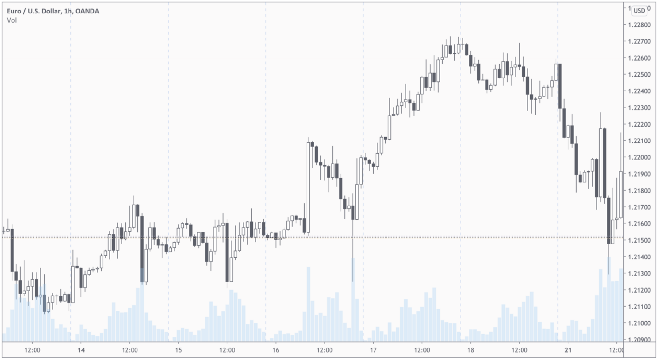How do I create a price candlestick + volume bar chart with shared x-axis?

I’m trying to recreate this blog post using the HoloViz tools. I would like to add it to awesome-panel.org and use it for work.

One of the things I cannot recreate isI don’t nescessarily have to overlay the two. But at least I would like the candlestick and bar plots to use the same x-axis. Such that zoom etc. is linked.

But I’m not able to.

I can get to this. But the x-axis are not linked

from datetime import timedelta
import yfinance as yf
import hvplot.pandas as pd
import holoviews as hv
import numpy as np
hv.extension('bokeh')
import pandas as pd

def _extract_raw_data(ticker="MSFT", period="6mo", interval="1d"):
extractor = yf.Ticker(ticker)
return extractor.history(period=period, interval=interval).reset_index()

def _transform_data(raw_data: pd.DataFrame):
data = raw_data[["Date", "Open", "High", "Low", "Close", "Volume"]].copy(deep=True).rename(columns={
"Date": "time",
"Open": "open",
"High": "high",
"Low": "low",
"Close": "close",
"Volume": "volume",
})
t_delta = timedelta(hours=1)
data['time_start'] = data.time - 9*t_delta # rectangles start
data['time_end'] = data.time + 9*t_delta    # rectangles end
data['positive'] = ((data.close - data.open)>0).astype(int)
return data

def make_candle_stick(data):
_delta = np.median(np.diff(data.time))
candlestick = hv.Segments(data, kdims=['time', 'low', 'time', 'high']) * hv.Rectangles(data, kdims=['time_start','open', 'time_end', 'close'], vdims=['positive'])
candlestick = candlestick.redim.label(Low='Values')
return candlestick.opts(hv.opts.Rectangles(color='positive', cmap=['red', 'green'], responsive=True), hv.opts.Segments(color='black', height=400, responsive=True))

def make_volume_plot(data):
return data.hvplot(x="time", y="volume", kind="line", responsive=True, height=200)

raw_data = _extract_raw_data()
data = _transform_data(raw_data=raw_data)

candle_stick_plot = make_candle_stick(data)
volume_plot = make_volume_plot(data)
import panel as pn
pn.pane.HoloViews((candle_stick_plot+volume_plot).cols(1), sizing_mode="stretch_both").servable()

If I change kind="bar" to kind="line" the two axis are linked. But everybody in Finance would expect to have a bar plot - not a line plot.

Agreed that this is very frustrating, the best you can probably do is use a hv.Histogram to represent the bars. It’s the Histogram is misnamed and should be called ContinuousBars or something better.

2 Likes

Thanks @philippjfr

I got it working by using hv.Histogram as you suggested. I had to use a Panel GridSpec to layout the plots.

Now I need to fix/ lock some of the axis, put in nicely formatted axis and set some sensible toolbar defaults.

But it’s already so nice. Will share when I have it finalized.

1 Like

Hello!
Can you show code for this beautiful example? I’m trying to do price candlestick with holoviews

1 Like

Live version is here Volume Profile Analysis (awesome-panel.org).